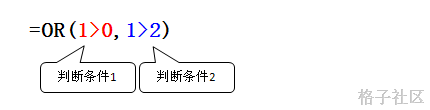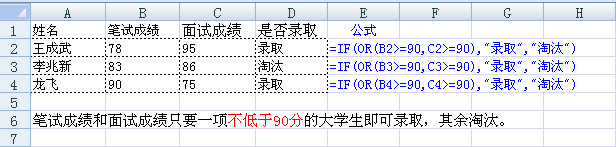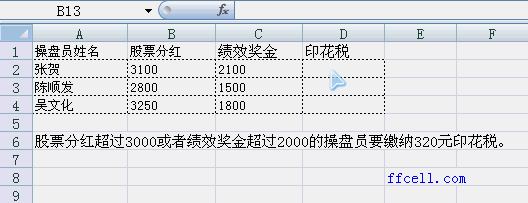`
Excel函数 教程

# Or函数##### 语法

`Or(判断条件1, 判断条件1,..., 判断条件255) `

1. 判断条件：是一个需要判断的表达式，也可以是数值。其结果只有2个:True（成立）或False（不成立）

## 例子

```=Or(1+1=2,5+5=3) 结果为True。第一个为True，第二个为False=Or(1+1=4,5+5=3) 结果为False。第一个为False，第二个为False=Or(1+1=1,5+5=3,1>9) 结果为False。全部为False
```